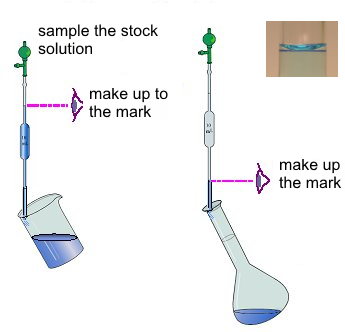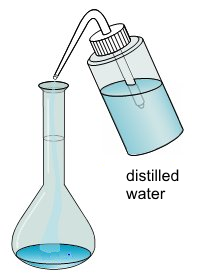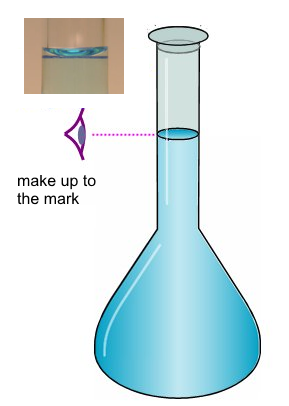Search:

# Preparation of a standard solution by dilution

- The volume of the mother solution that we want to dissolve in the given volume is calculated. For example, starting with one molar hydrochloric acid ($HCl_{conc }$) to prepare a $250 \;ml$ of a $0.04$ molar solution ($HCl_{dil}$), there will be needed: $n$ $=$ $[HCl_{dil}]\cdot V$ $=$ $0.04\cdot 0.25$ $=$ $0.01 \;mol\; HCl$, ie a volume: $v$ $=$ $\frac{n}{[HCl_{conc}]}$ $=$ $\frac{0,01}{1}$ $=$ $0.01 \;L$ $=$ $10\; ml \;HCl_{conc}$ - Use a pipette:- Rinse the pipette:- The required volume is taken:- Distilled water is filled in:- The calibration mark is targeted:- The flask is capped and stirred:- The standard solution (the molarity is known) is ready to use!

Images taken from: →   Lycée Clemenceau de Reims